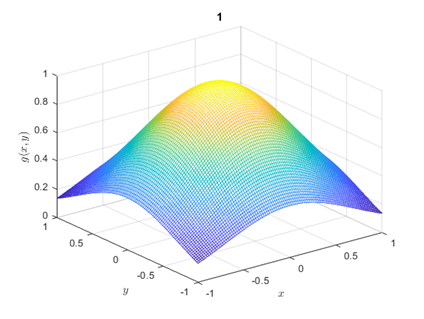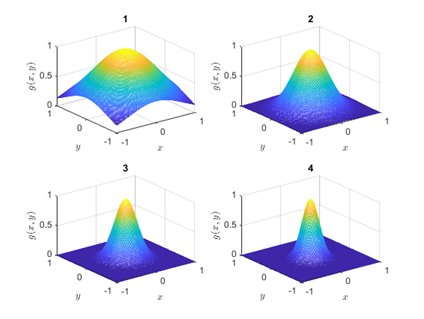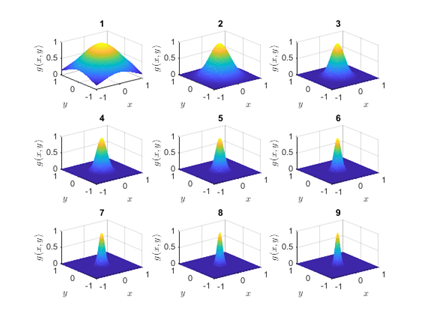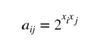# Introduction to Mathematical Software Assignment Help

We know that you are probably struggling with your mathematical software assignment and looking for a reliable MATLAB assignment tutor. Well, you do not need to search any further because we are the number one provider of introduction to mathematical software assignment help. We are an experienced website backed with a team of MATLAB veterans and former professors. Availing of our introduction to mathematical software homework help assures you of custom-written and error-free solutions within your deadline.

## MATLAB Function PlotsubI

% clear workpace and command window
clear, clc
% close all figures
close all
(a) Write a MATLAB function PlotsubI that takes a value as input and executes the following:
(i) Creates vectors and , each containing 101 points ranging from to .
(ii) Produces an subplot such that for each , its subwindow is occupied by a mesh plot of vs. and , where
(iii) Labels the , and axes of each mesh plot as , and respectively.
(b) Using the function PlotsubI, write suitable MATLAB commands in order to generate subplots in separate figure windows when n = 1, 2 and 3.

## MATLAB Function Code

function PlotsubI(n)
% Creates vectors x and y, each containing 101 points ranging from -1 to 1 .
x = linspace(-1, 1, 101) ;
y = linspace(-1, 1, 101) ;
% Produces an nxn subplot such that for each k,
figure ;
set(gcf,'NumberTitle','off')
set(gcf,'Name',['n=', num2str(n)])
for k = 1:n^2
% subplots nxn
subplot(n,n, k)
% mesh grid for X and Y
[X,Y] = meshgrid(x);
% compute g(x,y) as Z
Z = exp(-k^2 * (X.^2 + Y.^2) );
mesh(X,Y,Z)
xlabel('$x$','interpreter','latex')
ylabel('$y$','interpreter','latex')
zlabel('$g(x,y)$','interpreter','latex')
title(k)
end
end
% (b) Test the function for n = 1, 2 and 3
nlist = [1 2 3];
for i = 1: numel(nlist)
n = nlist(i) ;
% calls on function for each n
PlotsubI(n)
end## Expomat Functions that takes Vector X

Let be any 1 × m vector. The m × m expomatrix of x is a matrix denoted by(a) Write a MATLAB function Expomat that takes a vector x as input, and computes the corresponding expomatrix A.
function A = Expomat(x)
%EXPOMAT
% Expomat(x) that takes a vector x as input, and
% computes the corresponding expomatrix A
% find length of vector x
m = length(x);
% initialize a
a= zeros(m,m) ;
for i = 1: m
for j = 1:m
a(i,j) = 2^(x(i)*x(j)) ;
end
end
% output Matrix
A = a;
end
(b) Write and execute a MATLAB script Asum that uses Expomat with input vector x = (0.5, 0.52, 0.54, ..., 1.74, 1.76) and output matrix A to calculate the sum of the first 50 entries in the 17th column of A. This calculated sum must be obtained using a �for� loop and stored as output variable S.
% Script Asum
x = [0.5:0.02:1.76];
A = Expomat(x);
S = 0; % initialize the sum
% for loop
for i= 1:50
for j = 17
S = S + A(i,j) ;
end
end
fprintf('The Sum value is: %g', S)
The Sum value is: 88.9558

Related Topics